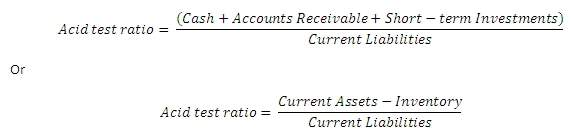# Acid Test Ratio

Posted in Finance, Accounting and Economics Terms, Total Reads: 6933

## Definition: Acid Test Ratio

The acid-test ratio is a measure to reflect whether the company has sufficient assets to meet its short-term liabilities without selling off its inventory. It is also referred to as quick ratio and its only difference from the current ratio is that inventories are removed from the current assets.

Mathematically, it is calculated as:

Formula:A value of over 1 suggests that the firm is in a position to meet its liabilities. A large variation of the acid test ratio from the current ratio implies that the assets of the firm are strongly dependent on the inventory.

Example

Assets Liabilities

Cash = 1000                                            Accounts payable = 500

Accounts receivable = 1000               Other short-term liabilities = 500

Inventory = 200

Current assets = 2200                     Current Liabilities = 1000

Acid test ratio = (2200-200)/1000 = 2

Hence, this concludes the definition of Acid Test Ratio along with its overview.

Browse the definition and meaning of more terms similar to Acid Test Ratio. The Management Dictionary covers over 7000 business concepts from 6 categories. This definition and concept has been researched & authored by our Business Concepts Team members.

Search & Explore : Management Dictionary

Similar Definitions from same Category: